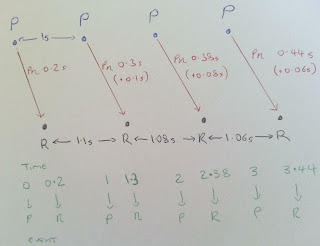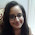## Thursday, 4 April 2013

### The Wenckebach Counter-Intuition

We are going to depart a little from our usual format today and address an ECG related question we asked yesterday.

This all stem's from this week's ECG which showed a Type I 2nd Degree AV block, a.k.a Wenckebach conduction.

Features of Wenckebach

So we know the features of Wenckebach conduction, which are:

• Progressive PR lengthening resulting in non-conducted P wave
• Progressive R-R interval shortening
• R-R interval length of dropped beat less than twice shortest R-R cycle
• Grouped beating
But let's stop for a second, the PR gets longer whilst the R-R gets shorter, that doesn't seem to make sense.

Why given the progressive PR prolongation do you get R-R interval shortening ?

Let's look at some of features of Wenckebach conduction again:
• Progressive PR lengthening
• BUT the magnitude of the lengthening progressively decreases
• The greatest increase in PR is from the 1st to 2nd beat following a dropped complex
• Regular P waves
• P-P interval is relatively fixed
We'll that has certainly cleared everything up !

This is quite a migraine inducing concept to think through and it made more sense when I went through a Wenckebach example.

Wenckebach Example

So let's assume:
• P wave occurs every second
• We have regular P wave activity with a relatively fixed P-P interval
• PR intervals increases by 0.1s then 0.08s then 0.06s
• We have progressive lengthening but with a decreasing magnitude
• Let's assume the first PR interval is 0.2s
• So the PR intervals are 0.2s -> 0.3s -> 0.38s -> 0.44s
• Now let's start our conduction at time 0 seconds

Time (s)      0         0.2         1        1.3         2         2.38        3       3.44        4

Event          P        QRS       P        QRS      P        QRS        P      QRS       P

• This means the R-R intervals are 1.1s -> 1.08s -> 1.06s
• Progressive shortening
• This is also the reason why the largest R-R interval is less than twice the shortest R-R interval, I'll let you do the math's on this one.
I found drawing a 'ladder diagram' helped me organise my thoughts so I scanned my scribbling and stuck it here.

﻿﻿Click to enlarge
﻿﻿You can see how ladder diagrams can be used at Nelson's EKG site here.

Christopher's response to our Wenckebach question

Christopher , a fellow ECG enthusiast and regular contributor to the comments section, posted an excellent explanation for our PR/RR puzzler. His comments can be found on the blog here, and I've pasted them below.

The amount the PR interval prolongs becomes less with each beat, leading to the shortening R-R interval (presuming a fixed P-P).

I found it to be an abstract concept to wrap my head around at first!

If you think of the R-R in terms of the PRi, it is a bit easier. With a fixed PRi you have a fixed R-R (we'll assume a fixed P-P). If you simply have a longer PRi the R-R does not change, and the same goes for a shorter PRi.

The PRi dictates when the R's occur relative to the P's.

If you vary the PRi from beat-to-beat, then you'll change when the R's occur from beat-to-beat. If you change when the R's occur, you'll change the R-R.

Going back, constant PRi's do not affect the R-R. Thus the amount which varies from beat-to-beat is the change in the R-R.

In AV Wenckebach the amount of beat-to-beat prolongation which occurs lessens (or stays the same), with the largest delta-PRi occurring in the first beat (e.g. 200ms, 260ms, 290ms, 310ms, drop; deltas of 60, 30, and 20). So, if the amount we vary decreases with each beat, the R-R will decrease with each beat.

Put in math form, assuming k is our baseline PRi and we have a repeating series of PRi's, where P is PP interval:

I hope that helps others understand it, I had to draw it a lot when I first noticed the decreasing R-R's.

#### 1 comment:

1.Excellent explanation! Thank you !!